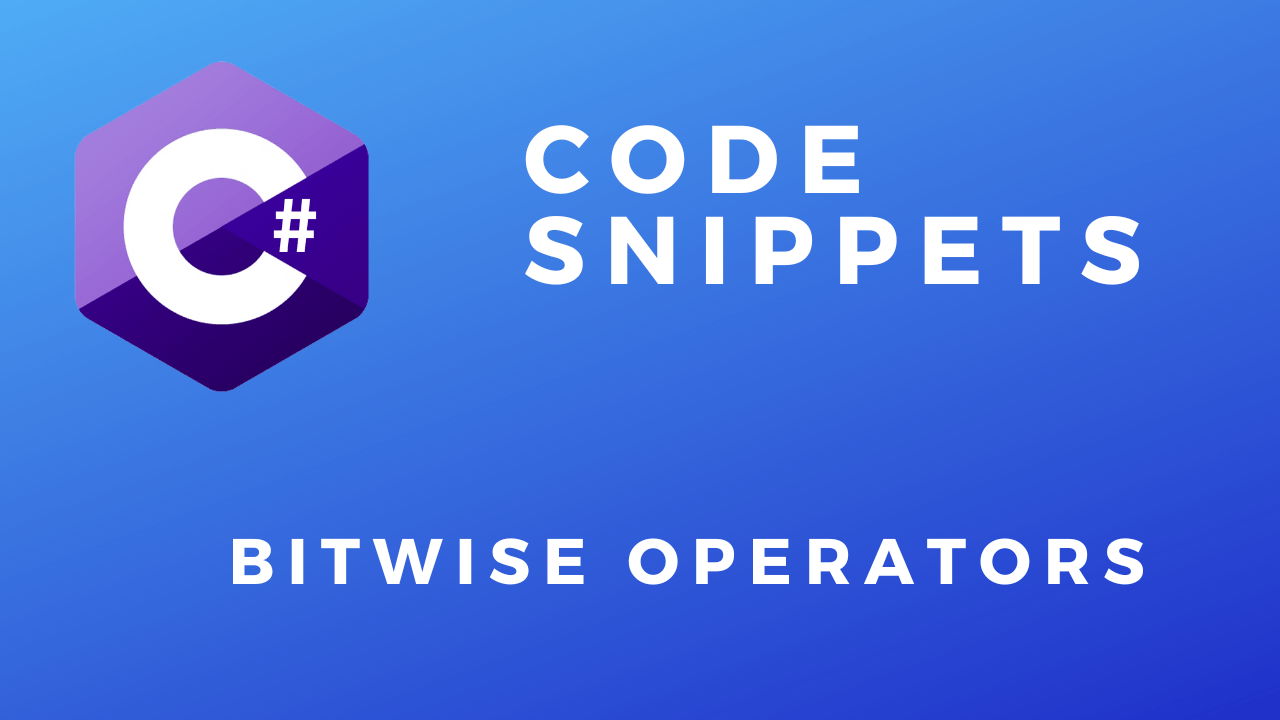# C# Bitwise OperatorsShare:

In this code snippetwe will take a look at bitwise operators in C#.

Bitwise operators can be used to manipulate individual bits of a variable. These operations can be quite cryptic and hard to understand compared to regular code. So only use it when it makes sense(for example bit masking) and don’t prematurely optimize your code. In C# we can use enums in conjunction with the Flags attribute to do bitmasking. This gives us cleaner and more readable code.

Here is a great video describing some of the things you can do with bit manipulation.

Let’s have a look at the code below to see how to use bitwise operators.

### Code:

In this example, I’ll demonstrate how to use the bitwise operators.
```int result = 0;

result = ALU(ALU_Operations.AND, 4, 2);
Console.WriteLine("AND: " + displayBinary(result));

result = ALU(ALU_Operations.OR, 4, 2);
Console.WriteLine("OR: " + displayBinary(result));

result = ALU(ALU_Operations.NOT, 4);
Console.WriteLine("NOT: " + displayBinary(result));

result = ALU(ALU_Operations.XOR, 4, 2);
Console.WriteLine("XOR: " + displayBinary(result));

result = ALU(ALU_Operations.LSR, 4, 2);
Console.WriteLine("LSR: " + displayBinary(result));

result = ALU(ALU_Operations.LSL, 4, 2);
Console.WriteLine("LSL: " + displayBinary(result));

//Using binary notation.
result = ALU(ALU_Operations.NOT, 0b0101);
Console.WriteLine("NOT 2: " + displayBinary(result));

//Let's make a simple arithmetical logical unit of a CPU.
static int ALU(ALU_Operations operation, int registerA, int registerB = 0)
{
int resultRegister = 0;

switch (operation)
{
case ALU_Operations.ADD: resultRegister = registerA + registerB;
break;
case ALU_Operations.SUB: resultRegister = registerA - registerB;
break;
case ALU_Operations.MUL: resultRegister = registerA * registerB;
break;
case ALU_Operations.DIV: resultRegister = registerA / registerB;
break;

//Bitwise operators
case ALU_Operations.AND: resultRegister = registerA & registerB; //AND register A with register B.
break;
case ALU_Operations.OR: resultRegister = registerA | registerB; //OR register A with register B.
break;
case ALU_Operations.NOT: resultRegister = ~registerA; //Invert register A.
break;
case ALU_Operations.XOR: resultRegister = registerA ^ registerB; //XOR register A with register B.
break;
case ALU_Operations.LSR: resultRegister = registerA >> registerB; //Shift right value in register A by number specified in register B.
break;
case ALU_Operations.LSL: resultRegister = registerA << registerB; //Shift left value in register A by number specified in register B.
break;
default: throw new NotSupportedException("Operation is not supported.");
break;
}

return resultRegister;
}

static string displayBinary(int number)
{
//Converts int to binary.
}

enum ALU_Operations
{
SUB,
MUL,
DIV,
AND,
OR,
NOT,
XOR,
LSR,
LSL
}```

### Resulting output:

In this next example, I’ll demonstrate one potential use case for bitwise operators which is bitmasking.
```//One thing we can do by manipulating bits with bitwise operators is bit masking.
//Applying a bit mask enables us to check weather a specific bit is set or not.
//A bool data type actually takes 1 byte of memory even though it only stores true/false.
//By using bitmasking with a byte/int data type we can use each bit as a true/false variable.
//This enables you to pack x8 more data in a byte/int variable than using a bool.

///////////////////////////////////// Note ////////////////////////////////////////////
//Usualy you don't need to go to such extreme memory saving masures.
//This process complicates you code. Use it only if you determine this would free a significat amount of memory.
//Also consider using a BitVector32 for this: https://learn.microsoft.com/en-us/dotnet/api/system.collections.specialized.bitvector32?view=net-7.0

//This is our data.
byte data = 211; //1101 0011

//Here we set which bit positions we want to mask out.
//For example, if we want to get the 5th bit from the data variable we need a mask like this:
byte mask = 16; //0001 0000

//Apply the mask using the & bitwise operator.
byte bit = (byte)(data & mask);

//Check the result.
Console.WriteLine("The 5th bit is set.");
else
Console.WriteLine("The 5th bit isn't set.");

//A more compact way to check a bit.
if ((data & 32) == 32) //The mask is 32 or 00010 0000 in binary.
Console.WriteLine("The 6th bit is set.");
else
Console.WriteLine("The 6th bit isn't set.");

//This is what happens with the bits:

//data:   1101 0011
//....... AND ....... Apply the AND logical opeartion.
//result: 0001 0000```

### Resulting output:

In this final example, I’ll demonstrate how to use enums with the Flags attribute to do bitmasking.
```//Select both Option1 and Option3.
Option selectedOptions = Option.Option1 | Option.Option3;

//Check selections.
if ((selectedOptions & Option.Option1) == Option.Option1)
Console.WriteLine("Option1 selected.");

if ((selectedOptions & Option.Option2) == Option.Option2)
Console.WriteLine("Option2 selected.");

if ((selectedOptions & Option.Option3) == Option.Option3)
Console.WriteLine("Option3 selected.");

if ((selectedOptions & Option.Option4) == Option.Option4)
Console.WriteLine("Option4 selected.");

//In C# we can use bitmasking in conjunction with enums.
[Flags]
enum Option
{
Option1 = 0, //Assign each enum a number that is the next power of two 0, 2, 4, 8, 16, 32, ...
Option2 = 2,
Option3 = 4,
Option4 = 8
}```

Share: